Most Affordable JEE | NEET | 8,9,10 Preparation by Kota's Top IITian Doctor Faculties

# NCERT Solutions for Class 11 Physics chapter 7 - System of Particles and Rotational Motion - Free PDF Download`
Hey, are you a class 11 student and looking for ways to download NCERT Solutions for Class 11 Physics chapter 7 "System of Particles and Rotational Motion"? If yes. Then read this post till the end.

In this article, we have listed NCERT solution for class 11 Physics Chapter 7 in PDF that are prepared by Kota’s top IITian’s Faculties by keeping Simplicity in mind.

If you want to learn and understand class 11 Physics chapter 7 "System of Particles and Rotational Motion" in an easy way then you can use these solutions PDF.

NCERT Solutions helps students to Practice important concepts of subjects easily. Class 11 Physics solutions provide detailed explanations of all the NCERT questions that students can use to clear their doubts instantly.

If you want to score high in your class 11 Physics Exam then it is very important for you to have a good knowledge of all the important topics, so to learn and practice those topics you can use eSaral NCERT Solutions.

So, without wasting more time Let’s start.

### Download The PDF of NCERT Solutions for Class 11 Physics Chapter 7 "System of Particles and Rotational Motion"

Question 1 Give the location of the centre of mass of a
(i) sphere,
(ii) cylinder,
(iii) ring, and
(iv) cube, each of uniform mass density. Does the centre of mass of a body necessarily lie inside the body?

Solution:
All the given objects have a uniform and symmetric mass density. Their centre of mass coincides with their geometric centres.
Centre of mass of an object need not to lie inside the body. For example, the centre of mass of a ring lies at its centre, which is outside the body.

Question 2. In the HCl molecule, the separation between the nuclei of the two atoms is about $1.27 \left(1 =10^{-10} \mathrm{~m}\right)$. Find the approximate location of the $\mathrm{CM}$ of the molecule, given that a chlorine atom is about $35.5$ times as massive as a hydrogen atom and nearly all the mass of an atom is concentrated in its nucleus.

Solution: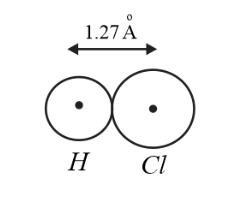Given,
The separation between the nuclei of $\mathrm{Cl}$ and $\mathrm{H}, d=1.27$
Let the mass of Hydrogen $m_{1}=m$
$\Rightarrow$ mass of the Chlorin $m_{2}=35.5 \mathrm{~m}$
For two particle system, the distance of the centre of mass from particle 1, i.e. Hydrogen is,
$r_{c m}=\frac{m_{2} d}{m_{1}+m_{2}}$
$r_{c m}=\frac{35.5 m \times 1.27}{m+35.5 m} =1.24$
$\therefore$ Centre of mass is located at a distance of $1.24$ from Hydrogen atom

Question 3 A child sits stationary at one end of a long trolley moving uniformly with a speed $V$ on a smooth horizontal floor. If the child gets up and runs about on the trolley in any manner, what is the speed of the $\mathrm{CM}$ of the (trolley + child) system?

Solution.
Speed of the centre of mass does not change. While the person is running randomly on the floor of the trolley, the force exerted by him on the trolley is a purely internal force of the system. Only external force causes the change in the velocity of the centre of mass. Hence the speed of the centre of mass is $V$.

Question.4. Show that the area of the triangle contained between the vectors $a$ and $b$ is one half of the magnitude of $a \times b$.

Solution.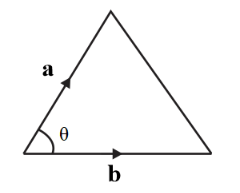Area of the triangle with adjacent sides $a$ and $b$,
$\Delta=\frac{1}{2} \times$ base $\times$ height
$\Delta=\frac{1}{2} \times b \times a \sin \theta$
From the definition of the cross product,
$b \times a \sin \theta=|a \times b|$
$\Rightarrow$ Area of the triangle $\Delta=\frac{1}{2}|a \times b|$

Question.5 Show that $a .(b \times c)$ is equal in magnitude to the volume of the parallelepiped formed on the three vectors, $a, b$ and $c$.

Solution.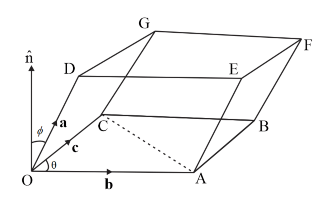From the given diagram, area of the base $O A B C=2 \times \frac{1}{2} \times b \times c=b \times c$
Height of the parallelepiped is component of $a$ perpendicular to the base $O A B C$, i.e. along $b \times c$.
Therefore, the height of the parallelepiped is $a \cos \phi$
$\therefore$ The volume of the parallelepiped $=a \times(b \times c) \cos \phi=a .(b \times c)$
Question.6 Find the components along the $x, y, z$ axes of the angular momentum $l$ of a particle, whose position vector is $r$ with components $x, y, z$ and momentum is $p$ with components $p_{x}, p_{y}$ and $p_{z} .$ Show that if the particle moves only in the $x$ - $y$ plane the angular momentum has only a $z$ -component.

Solution.
Given that the position vector $r=x \hat{\imath}+y \hat{\jmath}+z \hat{k}$ And momentum $p=p_{x} \hat{\imath}+p_{y} \hat{\jmath}+p_{z} \widehat{k}$
Angular momentum $l=r \times p=(x \hat{\imath}+y \hat{\jmath}+z \hat{k}) \times\left(p_{x} \hat{\imath}+p_{y} \hat{\jmath}+p_{z} \widehat{k}\right)$
$l=\left(y p_{z}-z p_{y}\right) \hat{\imath}+\left(z p_{x}-x p_{z}\right) \hat{\jmath}+\left(x p_{y}-y p_{x}\right) \hat{k}$
$\therefore$ Component of $l$ along $x$ -axis $=\left(y p_{z}-z p_{y}\right)$
Component of $l$ along $y$ -axis $=\left(z p_{x}-x p_{z}\right)$
Component of $l$ along $z$ -axis $=\left(x p_{y}-y p_{x}\right)$
If the particle moves only in the $x$ - $y$ plane, $z$ -component of the position vector and momentum are zero.
$\Rightarrow l=\left(y 0-0 p_{y}\right) \hat{\imath}+\left(0 p_{x}-x 0\right) \hat{\jmath}+\left(x p_{y}-y p_{x}\right) \hat{k}$
$\Rightarrow l=\left(x p_{y}-y p_{x}\right) \widehat{k}$
$\therefore$ If the particle moves only in the $x-y$ plane, angular momentum has only $z$ component.

Question.7 Two particles, each of mass $m$ and speed $v$, travel in opposite directions along parallel lines separated by a distance $d$. Show that the angular momentum vector of the two-particle system is the same whatever be the point about which the angular momentum is taken.

Solution.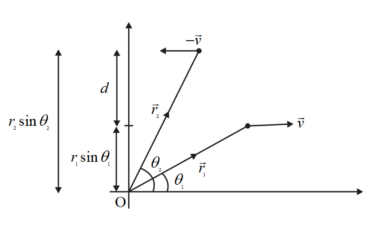Let the position vectors of the particle 1 and particle 2 are $\vec{r}_{1}$ and $\vec{r}_{2}$ respectively.
Their momenta can be written as $m \vec{v}$ and $-m \vec{v}$.
Angular momentum of the system $\vec{L}=\overrightarrow{r_{1}} \times m \vec{v}-r_{2} \times m \vec{v}$
$|\vec{L}|=m v r_{1} \sin \theta_{1}-m v r_{2} \sin \theta_{2}=m v\left(r_{1} \sin \theta_{1}-r_{2} \sin \theta_{2}\right)$
But from the diagram $\left(r_{1} \sin \theta_{1}-r_{2} \sin \theta_{2}\right)=d$
$\therefore$ irrespective of the reference point, the angular momentum of the system is always $m v d$

Question.8 A non-uniform bar of weight $\mathrm{W}$ is suspended at rest by two strings of negligible weight as shown in Fig.7.39. The angles made by the strings with the vertical are $36.9^{\circ}$ and $53.1^{\circ}$ respectively. The bar is $2 \mathrm{~m}$ long. Calculate the distance $d$ of the centre of gravity of the bar from its left end.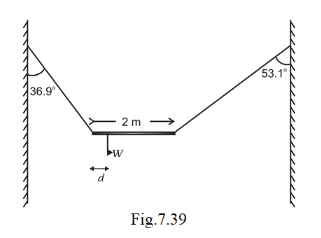Solution.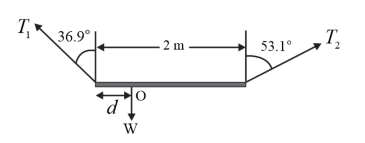As the bar is under equilibrium,
Sum of horizontal components of forces must be zero
$\Rightarrow T_{2} \sin 53.1^{\circ}-T_{1} \sin 36.9^{\circ}=0$
$\Rightarrow \frac{T_{1}}{T_{2}}=\frac{\sin 53.1^{\circ}}{\sin 36.9^{\circ}}=1.33$
And net torque about the centre of gravity must be zero
$\Rightarrow T_{1} \cos 36.9^{\circ} \times d-T_{2} \cos 53.1 \times(2-d)=0$
$\Rightarrow \frac{T_{1} \cos 36.9^{\circ}}{T_{2} \cos 53.1^{\circ}}=\frac{2-d}{d}$
$\Rightarrow 1.33 \times 1.33=\frac{2-d}{d}$
And
$\Rightarrow d=0.72 \mathrm{~m}=72 \mathrm{~cm}$
$\therefore$ The distance of the centre of gravity from the left end is $72 \mathrm{~cm}$.

Question.9 A car weighs $1800 \mathrm{~kg}$. The distance between its front and back axles is $1.8 \mathrm{~m}$. Its centre of gravity is $1.05 \mathrm{~m}$ behind the front axle. Determine the force exerted by the level ground on each front wheel and each back wheel.

Solution.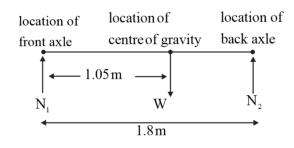Let, $N_{1}$ and $N_{2}$ are the net normal forces exerted by the level ground on the front and back wheels respectively.
As the car is in vertical equilibrium, the net vertical force must be zero.
$\Rightarrow N_{1}+N_{2}=W=1800 \mathrm{~g}=17640 \mathrm{~N}$
As the car is in rotational equilibrium, net torque about the centre of gravity must be zero.
$\Rightarrow N_{1} \times 1.05-N_{2} \times(1.8-1.05)=0$
$\Rightarrow N_{1}=\frac{0.75}{1.05} N_{2}=\frac{5}{7} N_{2}$
$\Rightarrow \frac{5}{7} N_{2}+N_{2}=17640$
$\Rightarrow N_{2}=10290 \mathrm{~N}$
And $N_{1}=7350 \mathrm{~N}$
$\therefore$ Force exerted by the level ground on each of the front wheels $=\frac{7350 \mathrm{~N}}{2}=$ $3675 \mathrm{~N}$
and that on the each of the back wheel $=\frac{10290 \mathrm{~N}}{2}=5145 \mathrm{~N}$

Question.10 (a) Find the moment of inertia of a sphere about a tangent to the sphere, given the moment of inertia of the sphere about any of its diameters to be $2 M R^{2} / 5$, where $M$ is the mass of the sphere and $R$ is the radius of the sphere.
(b) Given the moment of inertia of a disc of mass $M$ and radius $R$ about any of its diameters to be $M R^{2} / 4$, find its moment of inertia about an axis normal to the disc and passing through a point on its edge.

Solution.
Give that the moment of inertia of the sphere about any diameter $I_{c m}=\frac{2}{5} M R^{2}$
From parallel axis theorem
$I=I_{c m}+M d^{2}$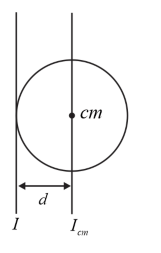Where $d$ is the distance between the required axis and the axis passing through the centre of mass.
$\Rightarrow d=R$
$I=\frac{2}{5} M R^{2}+M R^{2}=\frac{7}{5} M R^{2}$
Give that the moment of inertia about the diameter of the disc $=\frac{M R^{2}}{4}$
From the perpendicular axis theorem
$I_{z z}=I_{x x}+I_{y y}$
Where,
$I_{z z}, I_{x x}$ and $I_{y y}$ are the moment of inertia about three mutually perpendicular axes passing through a same point (centre of mass for this problem).
Also,
$I_{z z}$ is the moment of inertia about an axis perpendicular to the plane of the disc;
$I_{x x}$ and $I_{y y}$ are the moment of inertia about two mutually perpendicular axes lying on the plane of the disc.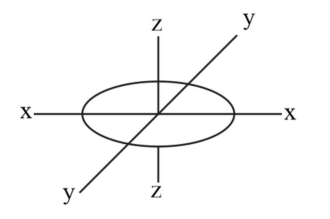$\Rightarrow I_{x x}=I_{y y}=\frac{M R^{2}}{4}$
$\Rightarrow I_{z z}=\frac{M R^{2}}{4}+\frac{M R^{2}}{4}=\frac{M R^{2}}{2}$
Thus, the moment of inertia of the disk about the axis passing through its centre of mass and perpendicular to the plane of the disc is $\frac{M R^{2}}{2}$.
$\Rightarrow I_{c m}=\frac{M R^{2}}{2}$
From the parallel axis theorem,
$I=I_{c m}+M d^{2}$
Where $d$ is the distance between the required axis and the axis passing through the centre of mass.
$\Rightarrow d=R$
$I=\frac{M R^{2}}{2}+M R^{2}=\frac{3}{2} M R^{2}$
$\therefore$ Moment of inertia about an axis perpendicular to the plane of the disc and passing through the edge of the disc $\frac{3}{2} M R^{2}$

Question.11 Torques of equal magnitude are applied to a hollow cylinder and a solid sphere, both having the same mass and radius. The cylinder is free to rotate about its standard axis of symmetry, and the sphere is free to rotate about an axis passing through its centre. Which of the two will acquire a greater angular speed after a given time.

Solution.
Given,
Mass of the hollow cylinder $=$ Mass of the solid sphere $=m$ (say)
The radius of the hollow cylinder $=$ The radius of the solid sphere $=R$ (say)
Moment of inertia of hollow cylinder about its axis $I_{C}=M R^{2}$
Moment of inertia of a solid sphere about the diameter $I_{S}=\frac{2}{5} M R^{2}$
From the torque and the angular acceleration relation,
$\tau=I \alpha$
For hollow cylinder
$\tau=M R^{2} \alpha_{C}$
$\alpha_{C}=\frac{\tau}{M R^{2}}$
Angular speed acquired by the cylinder in time $t$,
$\omega_{C}=0+\frac{1}{2} \alpha_{c} t^{2}=\frac{1}{2} \frac{\tau}{M R^{2}} t^{2}$
For solid sphere
$\tau=\frac{2}{5} M R^{2} \alpha_{C}$
$\alpha_{C}=\frac{\tau}{\frac{2}{5} M R^{2}}$
Angular speed acquired by the sphere in time $t$, $\omega_{S}=0+\frac{1}{2} \alpha_{c} t^{2}=\frac{1}{2} \frac{\tau}{\frac{2}{5} M R^{2}} t^{2}=\frac{5}{4} \frac{\tau}{M R^{2}} t^{2}$
$\Rightarrow$ Solid sphere acquires greater angular speed

Solution.12 A solid cylinder of mass $20 \mathrm{~kg}$ rotates about its axis with angular speed $100 \mathrm{rad} \mathrm{s}^{-1}$. The radius of the cylinder is $0.25 \mathrm{~m} .$ What is the kinetic energy associated with the rotation of the cylinder? What is the magnitude of angular momentum of the cylinder about its axis?

Solution.
Given,
Mass of the solid cylinder $M=20 \mathrm{~kg}$
Angular speed $\omega=100 \mathrm{rad} \mathrm{s}^{-1}$
The radius of the cylinder $R=0.25 \mathrm{~m}$
Rotational kinetic energy $K E=\frac{1}{2} I \omega^{2}$
$=\frac{1}{2} \frac{2}{5} M R^{2} \omega^{2}$
$=\frac{1}{2} \frac{1}{2} 20 \times 0.25^{2} \times 100^{2}$
$=3125 \mathrm{~J}$
Angular momentum $L=I \omega$
$=\frac{1}{2} 20 \times 0.25^{2} \times 100$
$=62.5 \mathrm{Js}$
Question.13 (a) A child stands at the centre of a turntable with his two arms outstretched. The turntable is set rotating with an angular speed of $40 \mathrm{rev} / \mathrm{min} .$ How much is the angular speed of the child if he folds his hands back and thereby reduces his moment of inertia to $2 / 5$ times the initial value? Assume that the turntable rotates without friction.

(b) Show that the child's new kinetic energy of rotation is more than the initial kinetic energy of rotation. How do you account for this increase in kinetic energy?

Solution.
Given that the initial angular speed of the turntable $\omega_{i}=40 \mathrm{rev} / \mathrm{min}$
Let the initial moment of inertia of the system is $I$
When the child folds his hands, system moment inertia becomes, $\frac{2}{5} I$
From the consecration of angular momentum,
Initial total angular momentum $=$ Final total angular momentum
$\Rightarrow I \times 40=\frac{2}{5} \times I \times \omega_{f}$
$\omega_{f}=100 \mathrm{rev} / \mathrm{min}$
The angular speed of the system after the child folding his hands is $100 \mathrm{rev} / \mathrm{min}$
Kinetic energy $K E=\frac{L^{2}}{2}$
As the angular momentum is conserved,
$K E \propto \frac{1}{x}$
$\Rightarrow \frac{\text { Initial kinetic energy }}{\text { Final kinetic energy }}=\frac{\frac{2}{5} I}{I}=\frac{2}{5}$
Final kinetic energy $=\frac{5}{2}$ Initial kinetic energy
The child uses his internal energy to increase the kinetic energy

Question.14 A rope of negligible mass is wound round a hollow cylinder of mass $3 \mathrm{~kg}$ and radius $40 \mathrm{~cm}$. What is the angular acceleration of the cylinder if the rope is pulled with a force of $30 \mathrm{~N}$ ? What is the linear acceleration of the rope? Assume that there is no slipping.

Solution.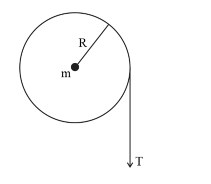Given,
Mass of the cylinder $m=3 \mathrm{~kg}$
The radius of the cylinder $R=40 \mathrm{~cm}=0.40 \mathrm{~m}$
Pulling force $=$ Tension in the rope $=30 \mathrm{~N}$
From torque and angular acceleration relation
$\tau=I \alpha$
$\Rightarrow 30 \times 0.40=3(0.40)^{2} \times \alpha$
$\Rightarrow \alpha=25 \mathrm{rad} \mathrm{s}^{-2}$
$\therefore$ Angular acceleration of the cylinder $\alpha=25 \mathrm{rad} \mathrm{s}^{-2}$
As the rope is not slipping on the cylinder
Acceleration of any particle on the rope $=$ liar acceleration of any particle on the rim of the cylinder
$\Rightarrow$ linear acceleration of the rope $a=R \alpha=0.40 \times 25=10 \mathrm{~m} \mathrm{~s}^{-2}$

Question.15 To maintain a rotor at a uniform angular speed of $200 \mathrm{rad} \mathrm{s}^{-1}$, an engine needs to transmit a torque of $180 \mathrm{~N} \mathrm{~m}$. What is the power required by the engine? (Note:uniform angular velocity in the absence of friction implies zero torque. In practice, applied torque is needed to counter frictional torque). Assume that the engine is $100 \%$ efficient.

Solution.
Given,
Uniform angular speed of the rotor $\omega=200 \mathrm{rad} \mathrm{s}^{-1}$
The torque required by the engine $\tau=180 \mathrm{~N} \mathrm{~m}$
Power required by the engine $P=\tau \times \omega=180 \times 200=36000 \mathrm{~W}=36 \mathrm{~kW}$

Question.16 From a uniform disk of radius $R$, a circular hole of radius $R / 2$ is cut out. The centre of the hole is at $R / 2$ from the centre of the original disc. Locate the centre of gravity of the resulting flat body.

Solution.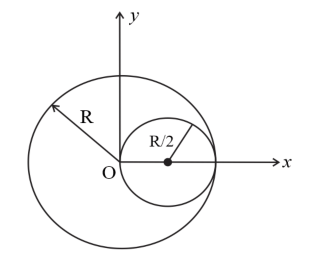Given that the radius of the disc $=R$
Let the mass of the whole disc be $M$
Centre of gravity of the removed portion and remaining portion system is at the geometric centre of the whole disc
Mass of the removed disc $m_{1}=\frac{M}{\pi R^{2}} \times \pi\left(\frac{R}{2}\right)^{2}=\frac{M}{4}$
Centre of mass coordinates of the removed disc are $\left(\frac{R}{2}, 0\right)$
Mass of the remaining portion $m_{2}=\frac{3 M}{4}$
Let the centre of mass coordinates of the remaining portion are $(x, 0)$
From the relation
$x_{c m}=\frac{m_{1} x_{1}+m_{2} x_{2}}{m_{1}+m_{2}}$
$\Rightarrow 0=\frac{\frac{M}{4} \times \frac{R}{2}+\frac{3 M}{4} \times x}{M}$
$\Rightarrow x=-\frac{R}{6}$
$\therefore$ Centre of gravity of the remaining body is at a distance of $\frac{R}{6}$ from the original centre, opposite to removed portion.

Question.17 A metre stick is balanced on a knife edge at its centre. When two coins, each of mass $5 \mathrm{~g}$ are put one on top of the other at the $12.0 \mathrm{~cm}$ mark, the stick is found to be balanced at $45.0 \mathrm{~cm}$. What is the mass of the metre stick?

Solution.
Given the length of the meter stick $L=1 \mathrm{~m}=100 \mathrm{~cm}$
Mass of each coin $m=5 \mathrm{~g}$
Let the mass of the meter stick be $M$
From the centre mass relation,
$x_{c m}=\frac{m_{1} x_{1}+m_{2} x_{2}}{m_{1}+m_{2}}$
$\Rightarrow 45.0 \mathrm{~cm}=\frac{((2 \times 5 \mathrm{~g}) \times 12 \mathrm{~cm})+(M \times 50 \mathrm{~cm})}{M+10 \mathrm{~g}}$
$\Rightarrow M=66 \mathrm{~g}$
$\therefore$ Mass of the meter stick is $66 \mathrm{~g}$.

Question.18 A solid sphere rolls down two different inclined planes of the same heights but different angles of inclination.
(a) Will it reach the bottom with the same speed in each case?
(b) Will it take longer to roll down one plane than the other?
(c) If so, which one and why?

Solution.
Let the mass of the solid sphere be $M$, and its radius be $R$
From the conservation of the energy
$M g H=\frac{1}{2} M v^{2}+\frac{1}{2} I \omega^{2}$
For the solid sphere rolling,
$I=\frac{2}{5} M R^{2}$ and $\omega=\frac{v}{R}$
$\Rightarrow M g H=\frac{1}{2} M v^{2}+\frac{1}{2} \frac{2}{5} M R^{2}\left(\frac{v}{R}\right)^{2}$
$\Rightarrow M g H=\frac{1}{2} M v^{2}+\frac{1}{5} M v^{2}=\frac{7}{10} M v^{2}$
$\Rightarrow v=\sqrt{\frac{10 \mathrm{gH}}{7}}$
As the height is the same for both planes, speeds with which the sphere reach the bottom will be the same
Acceleration of a body rolling down an inclined plane can be written as
$a=\frac{g \sin \theta}{\left(1+\frac{k^{2}}{R^{2}}\right)}$
$\Rightarrow a=\frac{g \sin \theta}{\left(1+\frac{2}{5}\right)}=\frac{5}{7} g \sin \theta$
$\Rightarrow$ Acceleration depends on $\sin \theta$
$\Rightarrow$ Distance to be travelled $S=H \operatorname{cosec} \theta$
$\Rightarrow H \operatorname{cosec} \theta=\frac{1}{2} \times \frac{5}{7} g \sin \theta t^{2}$
$\Rightarrow t=\sqrt{\frac{14 H}{5 g \sin ^{2} \theta}}$
$\Rightarrow t \propto \frac{1}{\sin \theta}$
Sphere takes more time to travel on the plane with lesser inclination.
Acceleration of a body rolling down an inclined plane can be written as
$a=\frac{g \sin \theta}{\left(1+\frac{K^{2}}{R^{2}}\right)}$
$\Rightarrow a=\frac{g \sin \theta}{\left(1+\frac{2}{5}\right)}=\frac{5}{7} g \sin \theta$
$\Rightarrow$ Acceleration depends on $\sin \theta$
$\Rightarrow$ Distance to be travelled $S=H \operatorname{cosec} \theta$
$\Rightarrow H \operatorname{cosec} \theta=\frac{1}{2} \times \frac{5}{7} g \sin \theta t^{2}$
$\Rightarrow t=\sqrt{\frac{14 H}{5 g \sin ^{2} \theta}}$
$\Rightarrow t \propto \frac{1}{\sin \theta}$
Sphere takes more time to travel on the plane with lesser inclination.

Question.19 A hoop of radius $2 \mathrm{~m}$ weighs $100 \mathrm{~kg} .$ It rolls along a horizontal floor so that its centre of mass has a speed of $20 \mathrm{~cm} / \mathrm{s}$. How much work has to be done to stop it?

Solution.
Given,
Mass of the hoop $m=100 \mathrm{~kg}$
The radius of the hoop $R=2 \mathrm{~m}$
Speed of the centre of mass $v_{c m}=20 \mathrm{~cm} / \mathrm{s}=0.20 \mathrm{~m} / \mathrm{s}$
The total energy of the hoop $E=\frac{1}{2} m v^{2}+\frac{1}{2} I \omega^{2}$
For rolling motion, $\omega=\frac{v}{R}$
$\Rightarrow E=\frac{1}{2} m v^{2}+\frac{1}{2}\left(m R^{2}\right)\left(\frac{v}{R}\right)^{2}$
$\Rightarrow E=m v^{2}=100 \times 0.20^{2}=4 \mathrm{~J}$
$\therefore$ Work required to stop the hoop $W=4 \mathrm{~J}$

Question.20 The oxygen molecule has a mass of $5.30 \times 10^{-26} \mathrm{~kg}$ and a moment of inertia of $1.94 \times 10^{-46} \mathrm{~kg} \mathrm{~m}^{2}$ about an axis through its centre perpendicular to the lines joining the two atoms. Suppose the mean speed of such a molecule in a gas is $500 \mathrm{~m} / \mathrm{s}$ and that its kinetic energy of rotation is two thirds of its kinetic energy of translation. Find the average angular velocity of the molecule.

Solution.
Given,
The mass of the oxygen molecule $m=5.30 \times 10^{-26} \mathrm{~kg}$ Moment of inertia $I=1.94 \times 10^{-46} \mathrm{~kg} \mathrm{~m}^{2}$
Mean speed $v=500 \mathrm{~m} / \mathrm{s}$
Translational kinetic energy $\quad K E_{T}=\frac{1}{2} m v^{2}=\frac{1}{2} \times 5.30 \times 10^{-26} \mathrm{~kg} \times$
$(500 \mathrm{~m} / \mathrm{s})^{2}$
$\Rightarrow K E_{T}=6.63 \times 10^{-21} \mathrm{~J}$
$\Rightarrow$ Rotational kinetic energy $K E_{R}=\frac{2}{3} K E_{T}=\frac{2}{3} \times 6.63 \times 10^{-21} \mathrm{~J}=4.42 \times$
$10^{-21} \mathrm{~J}$
$\Rightarrow K E_{R}=\frac{1}{2} I \omega^{2}=4.42 \times 10^{-21} \mathrm{~J}$
$\Rightarrow \omega=\sqrt{\frac{2 \times 4.42 \times 10^{-21}}{1.94 \times 10^{-46}}}=6.75 \times 10^{12} \mathrm{rad} \mathrm{s}^{-1}$
$\therefore$ The average angular velocity of the molecule is $6.75 \times 10^{12} \mathrm{rad} \mathrm{s}^{-1}$.

Solution.21 A solid cylinder rolls up an inclined plane of angle of inclination $30^{\circ} .$ At the bottom of the inclined plane the centre of mass of the cylinder has a speed of $5 \mathrm{~m} / \mathrm{s}$
(a) How far will the cylinder go up the plane?

Solution.
Given,
The linear speed of the cylinder at the bottom of the inclined plane $v=5 \mathrm{~m} \mathrm{~s}^{-1}$
The angle of inclination $\theta=30^{\circ}$
Let the height to which the cylinder can climb is $h$
From the conservation of mechanical energy,
$\frac{1}{2} m v^{2}+\frac{1}{2} I \omega^{2}=m g h$
$\Rightarrow \frac{1}{2} m v^{2}+\frac{1}{2} \times \frac{1}{2} m r^{2} \times\left(\frac{v}{r}\right)^{2}=m g h$
$\Rightarrow \frac{3 v^{2}}{4 g}=h$
$\Rightarrow$ Distance travelled on the inclined plane $d=h \operatorname{cosec} \theta=\frac{3 v^{2}}{4 g} \operatorname{cosec} \theta$
$d=\frac{3(5)^{2}}{4(9.8)} \operatorname{cosec} 30^{\circ}=3.8 \mathrm{~m}$
Acceleration of the cylinder while rolling down the inclined plane
$a=\frac{g \sin \theta}{\left(1+\frac{k^{2}}{r^{2}}\right)}=\frac{g \sin \theta}{\left(1+\frac{1}{2}\right)}=\frac{2}{3} g \sin \theta$
From the kinematic equation,
$S=u t+\frac{1}{2} a t^{2}$
$\Rightarrow 3.8 \mathrm{~m}=\frac{1}{2} \times \frac{2}{3} g \sin \theta \times t^{2}$
$\Rightarrow t=\sqrt{\frac{3 \times 3.8}{9.8 \times \frac{1}{2}}}=1.5 \mathrm{~s}$
Thus, total time taken to return to the bottom $=2 \times 1.5 \mathrm{~s}=3.0 \mathrm{~s}$

Question.22 As shown in Fig. 7.40, the two sides of a step ladder BA and CA are $1.6 \mathrm{~m}$ long and hinged at A. A rope DE, $0.5 \mathrm{~m}$ is tied halfway up. A weight $40 \mathrm{~kg}$ is suspended from a point $\mathrm{F}, 1.2 \mathrm{~m}$ from $\mathrm{B}$ along the ladder BA. Assuming the floor to be frictionless and neglecting the weight of the ladder, find the tension in the rope and forces exerted by the floor on the ladder. (Take $g=9.8 \mathrm{~m} / \mathrm{s}^{2}$ ) (Hint: Consider the equilibrium of each side of the ladder separately.)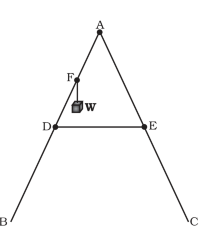Solution: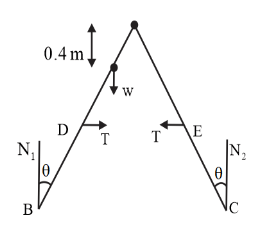Given,
Weight suspended $W=40 \mathrm{~g} \mathrm{~N}$
Length of the side $A B=A C=1.6 \mathrm{~m}$
Length of the rope $D E=0.5 \mathrm{~m}$
From the rules of triangles,
$B C=\frac{D E}{0.8} \times 1.6=1 \mathrm{~m}$
Let the angle between normal force and sides of the ladder is $\theta$
$\sin \theta=\frac{0.25}{0.8}=\frac{2.5}{8}$, and $\cos \theta=\frac{7.6}{8}$
Let the normal force applied by the floor on the ladder at $B$ and $C$ are $N_{1}$ and $N_{2}$ respectively.
As the ladder is under equilibrium,
$W=N_{1}+N_{2}=40 \mathrm{~g}$

Torque about $B$
$N_{1} \times 0+W \times 1.2 \sin \theta-N_{2}(1)+T(0.8 \cos \theta)-T(0.8 \cos \theta)=0$
$\Rightarrow N_{2}=\frac{40 \times 9.8 \times 1.2 \times 2.5}{8}=147 \mathrm{~N}$
And $N_{1}=40 \times 9.8-147=245 \mathrm{~N}$
Net torque on the left part of the ladder about $A$ is zero.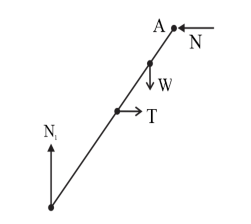$\Rightarrow N_{1} \times 1.6 \sin \theta-T \times 0.8 \cos \theta-W \times 0.4 \sin \theta=0$

$\Rightarrow 245 \times 1.6 \times \frac{2.5}{8}-T \times 0.8 \times \frac{7.6}{8}-40 \times 9.8 \times 0.4 \times \frac{2.5}{8}=0$
$\Rightarrow T=97 \mathrm{~N}$
Therefore, the tension in the rope $T=97 \mathrm{~N}$

Question.23 A man stands on a rotating platform, with his arms stretched horizontally holding a $5 \mathrm{~kg}$ weight in each hand. The angular speed of the platform is 30 revolutions per minute. The man then brings his arms close to his body with the distance of each weight from the axis changing from $90 \mathrm{~cm}$ to $20 \mathrm{~cm}$. The moment of inertia of the man together with the platform may be taken to be constant and equal to $7.6 \mathrm{~kg} \mathrm{~m}^{2}$.
(a) What is his new angular speed? (Neglect friction.)
(b) Is kinetic energy conserved in the process? If not, from where does the change come about?

Solution.
Given,
The initial angular speed of the platform $\omega_{i}=30$ revolutions per minute
The initial moment of inertial $I_{i}=7.6+2 \times 5 \times 0.9^{2}=15.7 \mathrm{~kg} \mathrm{~m}^{2}$
The final moment of inertia $I_{f}=7.6+2 \times 5 \times 0.2^{2}=8 \mathrm{~kg} \mathrm{~m}^{2}$
From the conservation of angular momentum principle,
$L_{i}=L_{f} \Rightarrow I_{i} \omega_{i}=I_{f} \omega_{f}$
$\Rightarrow \omega_{f}=\frac{I_{i}}{I_{f}} \omega_{i}=\frac{15.7}{8} \times 30$ revolutions per minute
$=59$ revolutions per minute
Therefore, new angular speed $\omega_{f}=59$ revolutions per minute
Kinetic energy $K E=\frac{L}{I}$
As the angular momentum is conserved, $K E \propto \frac{1}{I}$
As the moment of inertia is decreasing, kinetic energy increases in the process.
Increased energy comes from work done by the person.

Question.24 A bullet of mass $10 \mathrm{~g}$ and speed $500 \mathrm{~m} / \mathrm{s}$ is fired into a door and gets embedded exactly at the centre of the door. The door is $1.0 \mathrm{~m}$ wide and weighs $12 \mathrm{~kg}$. It is hinged at one end and rotates about a vertical axis practically without friction. Find the angular speed of the door just after the bullet embeds into it. (Hint: The moment of inertia of the door about the vertical axis at one end is $\left.\frac{M L^{2}}{3} .\right)$

Solution.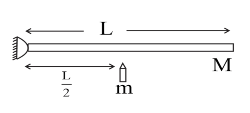Given,
The width of the door $L=1.0 \mathrm{~m}$
Mass of the door $M=12 \mathrm{~kg}$
Mass of the bullet $m=10 \mathrm{~g}=0.01 \mathrm{~kg}$
Speed of the bullet $v=500 \mathrm{~m} \mathrm{~s}^{-1}$
Moment of inertia of the door about one end $I=\frac{M L^{2}}{3}$
From the principle of conservation of angular momentum,
$m v \frac{L}{2}+0=\left(\frac{M L^{2}}{3}+m\left(\frac{L}{2}\right)^{2}\right) \omega$
$\omega=\frac{m v \frac{L}{2}}{\left(\frac{M L^{2}}{3}+m\left(\frac{L}{2}\right)^{2}\right)}=\frac{0.01 \times 500 \times \frac{1}{2}}{\frac{12(1)^{2}}{3}+0.01\left(\frac{1}{2}\right)^{2}}=0.625 \mathrm{rad} \mathrm{s}^{-1}$
Hence, the angular speed of the door just after the bullet embeds into it $0.625 \mathrm{rad} \mathrm{s}^{-1}$

Question.25 Two discs of moments of inertia $I_{1}$ and $I_{2}$ about their respective axes (normal to the disc and passing through the centre), and rotating with angular speeds $\omega_{1}$ and $\omega_{2}$ are brought into contact face to face with their axes of rotation coincident.
(a) What is the angular speed of the two-disc system?
(b) Show that the kinetic energy of the combined system is less than the sum of the initial kinetic energies of the two discs. How do your account for this loss in energy? Take $\omega_{1} \neq \omega_{2}$

Solution.
Given
The moment of inertia of the two disks are $I_{1}$ and $I_{2}$.
Initial angular velocities are $\omega_{1}$ and $\omega_{2}$.
From the conservation of the angular momentum
$I_{1} \omega_{1}+I_{2} \omega_{2}=\left(I_{1}+I_{2}\right) \omega$, where $\omega$ is the angular speed of the two-disc system.
$\Rightarrow \omega=\frac{I_{1} \omega_{1}+I_{2} \omega_{2}}{I_{1}+I_{2}}$
Initial total kinetic energy $K E_{i}=\frac{1}{2} I_{1} \omega_{1}^{2}+\frac{1}{2} I_{2} \omega_{2}^{2}$
Final total kinetic energy $K E_{f}=\frac{1}{2}\left(I_{1}+I_{2}\right) \omega^{2}=\frac{1}{2}\left(I_{1}+I_{2}\right)\left(\frac{I_{1} \omega_{1}+I_{2} \omega_{2}}{I_{1}+I_{2}}\right)^{2}$
$\Rightarrow K E_{i}-K E_{f}=\frac{1}{2} I_{1} \omega_{1}^{2}+\frac{1}{2} I_{2} \omega_{2}^{2}-\frac{1}{2}\left(I_{1}+I_{2}\right)\left(\frac{I_{1} \omega_{1}+I_{2} \omega_{2}}{I_{1}+I_{2}}\right)^{2}$
$\Rightarrow K E_{f}-K E_{i}=\frac{1}{2}\left(I_{1} \omega_{1}^{2}+I_{2} \omega_{2}^{2}-\frac{\left(I_{1} \omega_{1}\right)^{2}}{I_{1}+I_{2}}-\frac{\left(I_{2} \omega_{2}\right)^{2}}{I_{1}+I_{2}}-\frac{2 I_{1} \omega_{1} I_{2} \omega_{2}}{I_{1}+I_{2}}\right)$
$=\frac{1}{2\left(I_{1}+I_{2}\right)}\left(I_{1}^{2} \omega_{1}^{2}+I_{1} I_{2} \omega_{1}^{2}+I_{2}^{2} \omega_{2}^{2}+I_{1} I_{2} \omega_{2}^{2}-I_{1}^{2} \omega_{1}^{2}-I_{2}^{2} \omega_{2}^{2}-2 I_{1} I_{2} \omega_{1} \omega_{2}\right)$
$=\frac{I_{1} I_{2}}{2\left(I_{1}+I_{2}\right)}\left(\omega_{1}^{2}+\omega_{2}^{2}-2 \omega_{1} \omega_{2}\right)=\frac{I_{1} I_{2}\left(\omega_{1}-\omega_{2}\right)^{2}}{2\left(I_{1}+I_{2}\right)}$
All the terms in the above equation are positive.
$\Rightarrow K E_{f}-K E_{i}>0$
$\Rightarrow K E_{f}>K E_{i}$
Loss in the kinetic energy is due to work done by the frictional forces between the two-disc surfaces, which is responsible for the common angular velocity. However, as friction is internal force for the system, angular momentum remains conserved.

Question.26 (a) Prove the theorem of perpendicular axes. (Hint: Square of the distance of a point $(x, y)$ in the $x-y$ plane from an axis through the origin and perpendicular to the plane is $\left.x^{2}+y^{2}\right)$.
(b) Prove the theorem of parallel axes. (Hint: If the centre of mass of a system of $n$ particles is chosen to be the origin $\Sigma m_{i} r_{i}=0$ )

Solution.
Statement: Moment of inertia of a plane lamina about an axis perpendicular to its plane is equal to the sum of moment of inertia about two mutually perpendicular axes concurrent with perpendicular axis and lying in the plane of the body
Proof: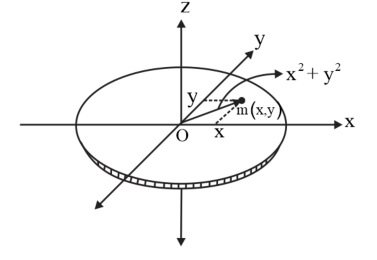Let the plane lamina is in $x-y$ plane, and we want to find moment of inertia about $z$ -axis.
Consider a particle of mass $m$ on the body with coordinates $(x, y)$
The distance of the particle from the $z$ -axis $r=\sqrt{x^{2}+y^{2}}$
Moment of inertia of the particle about $z$ -axis $I_{z}=m\left(x^{2}+y^{2}\right)=I_{y}+I_{x}$
$\Rightarrow$ for every particle on the plane lamina the relation $I_{z}=I_{y}+I_{x}$ holds true.
Thus, if $I_{x}$ is the moment of inertia of the lamina about $x$ -axis
$I_{y}$ is the moment of inertia of the lamina about $y$ -axis
And $I_{z}$ is the moment of inertia of the lamina about $z$ -axis
Then $I_{z}=I_{y}+I_{x}$
Statement: Moment of inertia of a body about a given axis is equal to the sum of moment of inertia about a parallel axis passing through its centre of mass and product of mass and square the distance between the two parallel axes.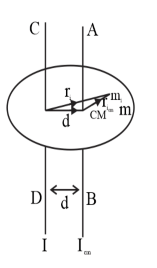Let $C D$ is the axis about which moment of inertia $I$ is required, and $A B$ is the parallel axis passing through the centre of mass.
For a small particle of mass $m_{i}$, with perpendicular distance $r_{i}$ from the $C D$ and perpendicular distance $r_{i, c m}$ as shown in figure
Moment of inertia about $C D$
$I=\Sigma m_{i} r_{i}^{2}$<
$r_{i}=r_{i, c m}+d$
$r_{i}^{2}=r_{i, c m}^{2}+d^{2}+2 r_{i, c m} \cdot d$
$\Rightarrow I=\Sigma m_{i} r_{i}^{2}=\Sigma m_{i} r_{i, c m}^{2}+\Sigma m_{i} d^{2}+\Sigma m_{i} 2 r_{i, c m} \cdot d$
$\Rightarrow I=\Sigma m_{i} r_{i}^{2}=\Sigma m_{i} r_{i, c m}^{2}+d^{2} \Sigma m_{i}+2\left(\Sigma m_{i} r_{i, c m}\right) \cdot d$
$\Rightarrow I=I_{c m}+M d^{2}+0\left(\because \Sigma m_{i} r_{i, c m}=0\right)$
Hence moment of inertia about given axis $C D, I=I_{c m}+M d^{2}$

Question.27 Prove the result that the velocity $v$ of translation of a rolling body (like a ring, disc, cylinder or sphere) at the bottom of an inclined plane of a height $h$ is given by $v^{2}=\frac{2 g h}{1+\frac{k^{2}}{R^{2}}}$using dynamical consideration (i.e. by consideration of forces and torques). Note $k$ is the radius of gyration of the body about its symmetry axis, and $R$ is the radius of the body. The body starts from rest at the top of the plane.

Solution:
Consider a rolling body of mass $m$ with radius of gyration $k$ is rolling on an inclined plane from a height $h$.
From the conservation of mechanical energy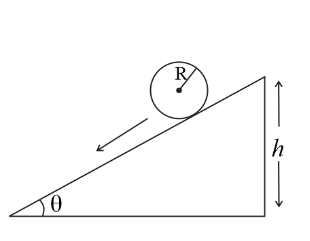$m g h=\frac{1}{2} m v^{2}+\frac{1}{2} I \omega^{2}$
$\Rightarrow m g h=\frac{1}{2} m v^{2}+\frac{1}{2} m k^{2}\left(\frac{v}{R}\right)^{2}$
$\Rightarrow v^{2}=\frac{2 g h}{1+\frac{k^{2}}{R^{2}}}$

Question.28 A disc rotating about its axis with angular speed $\omega_{o}$ is placed lightly (without any translational push) on a perfectly frictionless table. The radius of the disc is $R$. What are the linear velocities of the points $A, B$ and $C$ on the disc shown in Fig. 7.41? Will the disc roll in the direction indicated?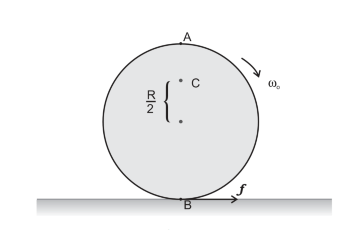Solution:
The linear velocity of point $A, v_{A}=R \times \omega_{o}$ towards the right
The linear velocity of the point $B, v_{B}=R \times \omega_{o}$ towards the left
The linear velocity of the point $C, v_{C}=\frac{R}{2} \times \omega_{o}$ towards the left
The disc cannot roll on a frictionless surface.

Question.29 Explain why friction is necessary to make the disc in Fig. $7.41$ roll in the direction indicated.
(a) Give the direction of frictional force at $\mathrm{B}$, and the sense of frictional torque, before perfect rolling begins.
(b) What is the force of friction after perfect rolling begins?

Solution.
Friction between point $B$ and the table pushes the disc in the forward direction. Without friction, there is no other force to set translational motion.
Friction force opposes the relative velocity of $B$ with respect to the table. Hence friction is in the same direction as the arrow.
The sense of the frictional torque is such that it opposes the angular speed $\omega_{o}$, i.e. anticlockwise sense. Its direction is perpendicular to the plane of the disc and coming out of the paper.
After perfect rolling begins the force of friction becomes zero. This is due to point $B$ (the point which is in contact with table) comes to rest momentarily during the perfect rolling motion.

Question.30 A solid disc and a ring, both of radius $10 \mathrm{~cm}$ are placed on a horizontal table simultaneously, with initial angular speed equal to $10 \pi \mathrm{rad} \mathrm{s}^{-1}$. Which of the two will start to roll earlier? The co-efficient of kinetic friction is $\mu_{k}=0.2$.

Solution.
Given,
Radii of solid disc and the ring $R=10 \mathrm{~cm}=0.1 \mathrm{~m}$
Initial angular speed $\omega_{o}=10 \pi \mathrm{rad} \mathrm{s}^{-1}$
Let the $m$ be the mass of the disc/ring, and $\omega$ be the final angular velocity after perfect rolling starts.
Also, the moment of inertia $I=m k^{2}$, where $k$ is the radius of gyration.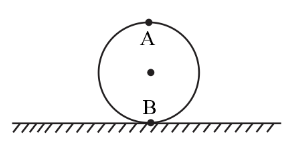As the net torque about the point $B$ is zero, angular momentum conserves about this point.
$L_{i}=L_{f} \Rightarrow I \omega_{o}=m R \omega R+I \omega$
$\Rightarrow \omega=\frac{I \omega_{o}}{m R^{2}+I}$
As the friction is kinetic, its magnitude is constant.
$\tau=-I \alpha$
$\alpha=-\frac{\tau}{I}=-\frac{\mu m g}{I}$
From the rotational kinematic equation
$\omega=\omega_{o}+\alpha t$
$t=-\frac{\omega-\omega_{o}}{\alpha}=\frac{\omega_{o}-\frac{I \omega_{o}}{m R^{2}+I}}{\frac{\mu m g}{I}}=\frac{I\left(m R^{2} \omega_{o}\right)}{\mu m g\left(m R^{2}+I\right)}=$
For ring
$t=\frac{m R^{2}\left(m R^{2} \omega_{o}\right)}{\mu m g\left(m R^{2}+m R^{2}\right)}=\frac{\left(R^{2} \omega_{o}\right)}{2 \mu g}=\frac{\left(0.1^{2} 10 \pi\right)}{2 \times 0.2 \times 9.8}=0.08 \mathrm{~s}$
For solid disc
$t=\frac{m R^{2}\left(m R^{2} \omega_{o}\right)}{2 \mu m g\left(m R^{2}+\frac{m R^{2}}{2}\right)}=\frac{\left(R^{2} \omega_{o}\right)}{3 \mu g}=\frac{\left(0.1^{2} 10 \pi\right)}{3 \times 0.2 \times 9.8}=0.05 \mathrm{~s}$
Hence the disc begins to roll early than the ring

Question.31 A cylinder of mass $10 \mathrm{~kg}$ and radius $15 \mathrm{~cm}$ is rolling perfectly on a plane of inclination $30^{\circ} .$ The coefficient of static friction $\mu_{s}=0.25$
(a) How much is the force of friction acting on the cylinder?
(b) What is the work done against friction during rolling?
(c) If the inclination $\theta$ of the plane is increased, at what value of $\theta$ does the cylinder begin to skid, and not roll perfectly?

Solution:
Given,
Mass of the cylinder $m=10 \mathrm{~kg}$
Radius $R=15 \mathrm{~cm}=0.15 \mathrm{~m}$
The inclination of the inclined plane $\theta=30^{\circ}$
Coefficient of the static friction $\mu_{s}=0.25$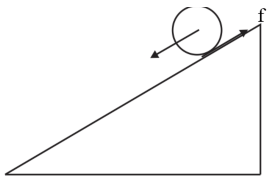Assuming that the cylinder is rolling down the inclined plane
Net force along the inclined plane
$m g \sin \theta-f=m a \ldots \ldots \ldots \ldots$ (i)
For a body rolling on inclined plane $a=\frac{g \sin \theta}{1+\frac{k^{2}}{R^{2}}}$
For solid cylinder $k=\sqrt{\frac{1}{2}} R$ $\Rightarrow a=\frac{2 g \sin \theta}{3}$
From equation (i)
$m g \sin \theta-f=\frac{m 2 g \sin \theta}{3}$
$f=\frac{m g \sin \theta}{3}=\frac{10 \times 9.8 \times \frac{1}{2}}{3}=16.3 \mathrm{~N}$
Hence friction force acting on the sphere $f=16.3 \mathrm{~N}$
As the point of application of friction is not moving instantly, work done by friction is zero.
Friction force $f=\frac{m g \sin \theta}{3}$
Also, the maximum possible friction force $f_{\max }=\mu m g \cos \theta$
$\Rightarrow \frac{m g \sin \theta}{3} \leq \mu m g \cos \theta$
$\Rightarrow \tan \theta \leq 3 \times 0.25$
$\Rightarrow \theta \leq 36.9^{\circ}$
If the angle of inclination is greater than $37^{\circ}$ (approximately) cylinder begins to skid on the inclined plane.

Solution.32 Read each statement below carefully, and state, with reasons, if it is true or false;
(a) During rolling, the force of friction acts in the same direction as the direction of motion of the CM of the body.
(b) The instantaneous speed of the point of contact during rolling is zero.
(c) The instantaneous acceleration of the point of contact during rolling is zero.
(d) For perfect rolling motion, work done against friction is zero.
(e) A wheel moving down a perfectly frictionless inclined plane will undergo slipping (not rolling) motion.

Solution.
False
During the rolling motion down the inclined plane, friction acts along the inclined plane upwards, whereas, the centre of mass moves along the inclined plane downwards.
True
In combined translation and rotation, the velocity of any particle is the vector sum of translation velocity and the velocity due to the rotation. In pure rolling, for the point of contact, these two velocities are equal and opposite. Hence, net velocity is zero.
False
If the acceleration of the point of contact is zero, for pure rolling motion, the point of contact must be at rest permanently, which is not true. Point of contact comes to rest momentarily. Hence acceleration of the point of contact during rolling motion is not zero.
True
For perfect rolling motion, the point of contact is momentarily at rest. Also, the force of friction is applied at this point. Hence work done by the friction, in this case, is zero.
True
While moving down the inclined plane, friction provides necessary torque for the rotation. Without friction wheel slides on the inclined plane.

Question.33 Separation of Motion of a system of particles into motion of the centre of mass and motion about the centre of mass:
(a) Show $p_{i}=p_{i}^{\prime}+m_{i} V$
where $p_{i}$ is the momentum of the $i^{t h}$ particle (of mass $m_{i}$ ) and $p_{i}^{\prime}=m_{i} v_{i}^{\prime}$. Note $v_{i}^{\prime}$ is the velocity of the $i^{t h}$ particle relative to the centre of mass.
Also, prove using the definition of the centre of mass $\sum p_{i}^{\prime}=0$
(b) Show $K=K^{\prime}+\frac{1}{2} M V^{2}$
where $K$ is the total kinetic energy of the system of particles, $K^{\prime}$ is the total kinetic energy of the system when the particle velocities are taken with respect to the centre of mass and $\frac{1}{2} M V^{2}$ is the kinetic energy of the translation of the system as a whole (i.e. of the centre of mass motion of the system). The result has been used in Sec. 7.14.
(c) Show $L=L^{\prime}+R \times M V$
where $L^{\prime}=\Sigma r_{i}^{\prime} \times p_{i}^{\prime}$ is the angular momentum of the system about the centre of mass with velocities taken relative to the centre of mass. Remember $r_{i}^{\prime}=r_{i}-R ;$ rest of the notation is the standard notation used in the chapter. Note $L^{\prime}$ and $M \times R V$ can be said to be angular momenta, respectively, about and of the centre of mass of the system of particles.
(d) Show $\frac{d L^{\prime}}{d t}=\Sigma r_{i}^{\prime} \times \frac{d p^{\prime}}{d t}$
Further, show that
$\frac{d L^{\prime}}{d t}=\tau_{e x t}^{\prime}$
where $\tau_{e x t}^{\prime}$ is the sum of all external torques acting on the system about the centre of mass. (Hint: Use the definition of centre of mass and third law of motion. Assume the internal forces between any two particles act along the line joining the particles.)

Solution:
Given,
Momentum of the $i^{t h}$ particle $=p_{i}=m_{i} v_{i}$
Velocity of the $i^{t h}$ particle relative to the centre of mass $=v_{i}^{\prime}$
Momentum of the $i^{t h}$ particle relative to the centre of mass $p_{i}^{\prime}=m_{i} v_{i}^{\prime}$
Let the velocity of the centre of mass with respect to the ground $=V$
Velocity of the $i^{t h}$ particle relative to the centre of mass $v_{i}^{\prime}=v_{i}-V$
$\Rightarrow$ Velocity of the $i^{t h}$ particle with respect to the ground frame $v_{i}=v_{i}^{\prime}+V$\
Multiplying with the mass of the $i^{t h}$ particle,
$m_{i} v_{i}=m_{i} v_{i}^{\prime}+m_{i} V$
$\Rightarrow p_{i}=p_{i}^{\prime}+m_{i} V$
Let the distance of the $i^{t h}$ particle from the centre of mass be $r_{i}^{\prime}$
$\Sigma p_{i}^{\prime}=\Sigma m_{i} v_{i}^{\prime}=\Sigma m_{i} \frac{d r_{i}^{\prime}}{d t}=\frac{d}{d t} \Sigma m_{i} r_{i}^{\prime}$
From the definition, $\Sigma m_{i} r_{i}^{\prime}=0$
$\Rightarrow \Sigma p_{i}^{\prime}=0$
Given,
The total kinetic energy of the system of particles $=K$
The total kinetic energy of the system of particles with respect to ground $=K^{\prime}$
The translational kinetic energy of the whole system $=\frac{1}{2} M V^{2}$
Velocity of the $i^{t h}$ particle relative to the centre of mass $v_{i}^{\prime}=v_{i}-V$
$\Rightarrow v_{i}=v_{i}^{\prime}+V$
The kinetic energy of the $i^{t h}$ particle $K_{i}=\frac{1}{2} m_{i}\left(v_{i} \cdot v_{i}\right)=\frac{1}{2} m_{i}\left\{\left(v_{i}^{\prime}\right)^{2}+V^{2}+\right.$
$\left.\left(v_{i}^{\prime} . V\right)\right\}$
The total kinetic energy of the system of particles $K=\Sigma K_{i}$ $\Rightarrow K=\Sigma\left[\frac{1}{2} m_{i}\left\{\left(v_{i}^{\prime}\right)^{2}+V^{2}+\left(v_{i}^{\prime} \cdot V\right)\right\}\right]=K^{\prime}+\frac{1}{2} M V^{2}+\frac{1}{2}\left(\Sigma m_{i} v_{i}^{\prime}\right) \cdot V$
But $\Sigma m_{i} v_{i}^{\prime}=0$
$\Rightarrow K=K^{\prime}+\frac{1}{2} M V^{2}$
Given
Angular momentum of the system about the centre of mass $=L^{\prime}=\Sigma r_{i}^{\prime} \times p_{i}^{\prime}$
Position of the $i^{t h}$ particle with respect to the centre of mass $r_{i}^{\prime}=r_{i}-R$
$r_{i}=r_{i}^{\prime}+R$
$\Rightarrow$ Velocity of the $i^{t h}$ particle $v_{i}=v_{i}^{\prime}+V$
Angular momentum of the $i^{t h}$ particle $L_{i}=m_{i}\left(r_{i} \times v_{i}\right)=m_{i}\left(r_{i}^{\prime}+R\right) \times\left(v_{i}^{\prime}+\right.$ $V$ )
$\Rightarrow L_{i}=m_{i}\left(r_{i}^{\prime} \times v_{i}^{\prime}+r_{i}^{\prime} \times V+R \times v_{i}^{\prime}+R \times V\right)$
Net angular momentum
$L=\Sigma L_{i}=\Sigma m_{i}\left(r_{i}^{\prime} \times v_{i}^{\prime}\right)+\Sigma m_{i}\left(r_{i}^{\prime} \times V\right)+\sum m_{i}\left(R \times v_{i}^{\prime}\right)+\sum m_{i}(R \times V)$
But $\sum m_{i}\left(r_{i}^{\prime} \times V\right)=\left(\Sigma m_{i} r_{i}^{\prime}\right) \times V=0$
And $\Sigma m_{i}\left(R \times v_{i}^{\prime}\right)=R \times\left(\Sigma m_{i} v_{i}^{\prime}\right)=0$
$\Rightarrow L=L^{\prime}+R \times M V$
$L^{\prime}=\Sigma m_{i}\left(r_{i}^{\prime} \times v_{i}^{\prime}\right)=\Sigma r_{i}^{\prime} \times p_{i}^{\prime}$
$\Rightarrow \frac{d L^{\prime}}{d t}=\Sigma\left(\frac{d}{d t} r_{i}^{\prime}\right) \times p_{i}^{\prime}+\Sigma r_{i}^{\prime} \times\left(\frac{d}{d t} p_{i}^{\prime}\right)$
$\Rightarrow \frac{d L^{\prime}}{d t}=\Sigma v_{i}^{\prime} \times p_{i}^{\prime}+\Sigma r_{i}^{\prime} \times\left(\frac{d}{d t} p_{i}^{\prime}\right)$
But $\sum v_{i}^{\prime} \times p_{i}^{\prime}=0\left(\because\right.$ The angle between $v_{i}^{\prime}$ and $p_{i}^{\prime}$ is zero)
$\Rightarrow \frac{d L^{\prime}}{d t}=\Sigma r_{i}^{\prime} \times\left(\frac{d}{d t} p_{i}^{\prime}\right)$
We have $\frac{d L^{\prime}}{d t}=\Sigma r_{i}^{\prime} \times\left(\frac{d}{d t} p_{i}^{\prime}\right)$
$\Rightarrow \frac{d L^{\prime}}{d t}=\Sigma r_{i}^{\prime} \times m_{i}\left(\frac{d}{d t} v_{i}^{\prime}\right)$
$\Rightarrow \frac{d L^{\prime}}{d t}=\Sigma r_{i}^{\prime} \times m_{i}\left(a_{i}^{\prime}\right)$
Here $m_{i} a_{i}^{\prime}$ is the net force and $r_{i}^{\prime} \times m_{i}\left(a_{i}^{\prime}\right)$ is the net torque acting on the $i^{t h}$ particle,
As the net torque due to internal forces adds up to zero $\Rightarrow \frac{d L^{\prime}}{d t}=\tau_{e x t}^{\prime}$